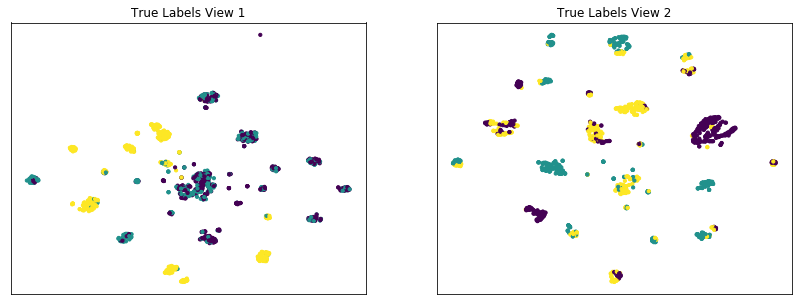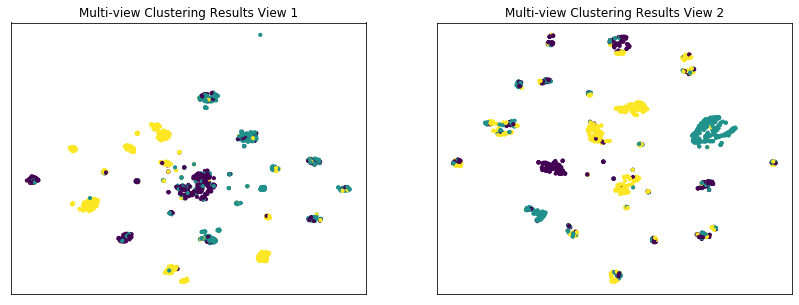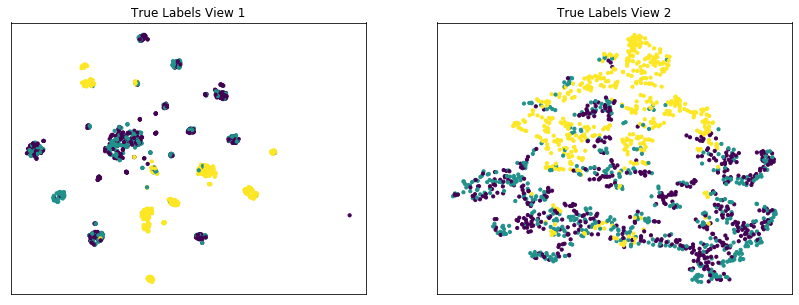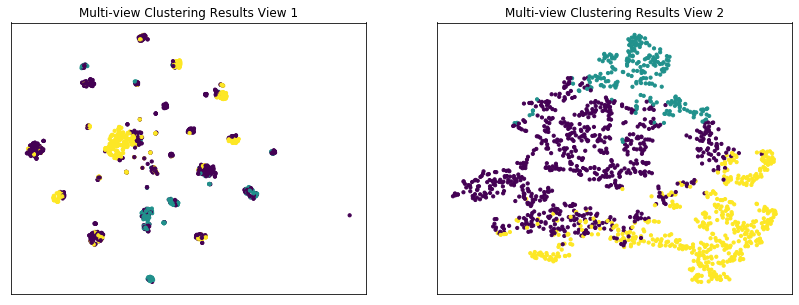# Assessing the Conditional Independence Views Requirement of Multi-view KMeans¶

In the following experiments, we will perform single-view kmeans clustering on the two views separately and on them concatenated together. We also perform multi-view clustering using the multi-view algorithm. We will also compare the performance of multi-view and single-view versions of kmeans clustering. We will evaluate the purity of the resulting clusters from each algorithm with respect to the class labels using the normalized mutual information metric.

:

import numpy as np
from numpy.random import multivariate_normal
import scipy as scp
from mvlearn.cluster.mv_k_means import MultiviewKMeans
from sklearn.metrics import normalized_mutual_info_score as nmi_score
from sklearn.cluster import KMeans
from sklearn.datasets import fetch_covtype
import matplotlib.pyplot as plt
%matplotlib inline
from sklearn.manifold import TSNE
import warnings
warnings.filterwarnings("ignore")
RANDOM_SEED=10


## Artificial dataset with conditionally independent views¶

Here, we create an artificial dataset where the conditional independence assumption between views, given the true labels, is enforced. Our artificial dataset is derived from the forest covertypes dataset from the scikit-learn package. This dataset is comprised of 7 different classes, with with 54 different numerical features per sample. To create our artificial data, we will select 500 samples from each of the first 6 classes in the dataset, and from these, construct 3 artificial classes with 2 views each.

:

def get_ci_data(num_samples=500):

#Load in the vectorized news group data from scikit-learn package
cov = fetch_covtype()
all_data = np.array(cov.data)
all_targets = np.array(cov.target)

#Set class pairings as described in the multiview clustering paper
view1_classes = [1, 2, 3]
view2_classes = [4, 5, 6]

#Create lists to hold data and labels for each of the classes across 2 different views
labels =  [num for num in range(len(view1_classes)) for _ in range(num_samples)]
labels = np.array(labels)
view1_data = list()
view2_data = list()

#Randomly sample items from each of the selected classes in view1
for class_num in view1_classes:
class_data = all_data[(all_targets == class_num)]
indices = np.random.choice(class_data.shape, num_samples)
view1_data.append(class_data[indices])
view1_data = np.concatenate(view1_data)

#Randomly sample items from each of the selected classes in view2
for class_num in view2_classes:
class_data = all_data[(all_targets == class_num)]
indices = np.random.choice(class_data.shape, num_samples)
view2_data.append(class_data[indices])
view2_data = np.concatenate(view2_data)

#Shuffle and normalize vectors
shuffled_inds = np.random.permutation(num_samples * len(view1_classes))
view1_data = np.vstack(view1_data)
view2_data = np.vstack(view2_data)
view1_data = view1_data[shuffled_inds]
view2_data = view2_data[shuffled_inds]
magnitudes1 = np.linalg.norm(view1_data, axis=0)
magnitudes2 = np.linalg.norm(view2_data, axis=0)
magnitudes1[magnitudes1 == 0] = 1
magnitudes2[magnitudes2 == 0] = 1
magnitudes1 = magnitudes1.reshape((1, -1))
magnitudes2 = magnitudes2.reshape((1, -1))
view1_data /= magnitudes1
view2_data /= magnitudes2
labels = labels[shuffled_inds]
return [view1_data, view2_data], labels


:

def perform_clustering(seed, m_data, labels, n_clusters):
#################Single-view kmeans clustering#####################
# Cluster each view separately
s_kmeans = KMeans(n_clusters=n_clusters, random_state=seed, n_init=100)
s_clusters_v1 = s_kmeans.fit_predict(m_data)
s_clusters_v2 = s_kmeans.fit_predict(m_data)

# Concatenate the multiple views into a single view
s_data = np.hstack(m_data)
s_clusters = s_kmeans.fit_predict(s_data)

# Compute nmi between true class labels and single-view cluster labels
s_nmi_v1 = nmi_score(labels, s_clusters_v1)
s_nmi_v2 = nmi_score(labels, s_clusters_v2)
s_nmi = nmi_score(labels, s_clusters)
print('Single-view View 1 NMI Score: {0:.3f}\n'.format(s_nmi_v1))
print('Single-view View 2 NMI Score: {0:.3f}\n'.format(s_nmi_v2))
print('Single-view Concatenated NMI Score: {0:.3f}\n'.format(s_nmi))

#################Multi-view kmeans clustering######################

# Use the MultiviewKMeans instance to cluster the data
m_kmeans = MultiviewKMeans(n_clusters=n_clusters, n_init=100, random_state=seed)
m_clusters = m_kmeans.fit_predict(m_data)

# Compute nmi between true class labels and multi-view cluster labels
m_nmi = nmi_score(labels, m_clusters)
print('Multi-view NMI Score: {0:.3f}\n'.format(m_nmi))

return m_clusters

:

def display_plots(pre_title, data, labels):

# plot the views
plt.figure()
fig, ax = plt.subplots(1,2, figsize=(14,5))
dot_size=10
ax.scatter(new_data[:, 0], new_data[:, 1],c=labels,s=dot_size)
ax.set_title(pre_title + ' View 1')
ax.axes.get_xaxis().set_visible(False)
ax.axes.get_yaxis().set_visible(False)

ax.scatter(new_data[:, 0], new_data[:, 1],c=labels,s=dot_size)
ax.set_title(pre_title + ' View 2')
ax.axes.get_xaxis().set_visible(False)
ax.axes.get_yaxis().set_visible(False)

plt.show()


## Comparing the performance of multi-view and single-view KMeans on our dataset with conditionally independent views¶

The co-Expectation Maximization framework (and co-training), relies on the fundamental assumption that data views are conditionally independent. If all views are informative and conditionally independent, then Multi-view KMeans is expected to produce higher quality clusters than Single-view KMeans, for either view or for both views concatenated together. Here, we will evaluate the quality of clusters by using the normalized mutual information metric, which is essentially a measure of the purity of clusters with respect to the true underlying class labels.

As we see below, Multi-view KMeans produces clusters with higher purity than Single-view KMeans across a range of values for the n_clusters parameter for data with complex and informative views, which is consistent with some of the results from the original Multi-view clustering paper.

:

data, labels = get_ci_data()
m_clusters = perform_clustering(RANDOM_SEED, data, labels, 3)

# Running TSNE to display clustering results via low dimensional embedding
tsne = TSNE()
new_data = list()
new_data.append(tsne.fit_transform(data))
new_data.append(tsne.fit_transform(data))
display_plots('True Labels', new_data, labels)
display_plots('Multi-view Clustering Results', new_data, m_clusters)

Single-view View 1 NMI Score: 0.342

Single-view View 2 NMI Score: 0.503

Single-view Concatenated NMI Score: 0.422

Multi-view NMI Score: 0.530


<Figure size 432x288 with 0 Axes><Figure size 432x288 with 0 Axes>## Artificial dataset with conditionally dependent views¶

Here, we create an artificial dataset where the conditional independence assumption between views, given the true labels, is violated. We again derive our dataset from the forest covertypes dataset from sklearn. However, this time, we use only the first 3 classes of the dataset, which will correspond to the 3 clusters for view 1. To produce view 2, we will apply a simple nonlinear transformation to view 1 using the logistic function, and we will apply a negligible amount of noise to the second view to avoid convergence issues. This will result in a dataset where the correspondance between views is very high.

:

def get_cd_data(num_samples=500):

#Load in the vectorized news group data from scikit-learn package
cov = fetch_covtype()
all_data = np.array(cov.data)
all_targets = np.array(cov.target)

#Set class pairings as described in the multiview clustering paper
view1_classes = [1, 2, 3]
view2_classes = [4, 5, 6]

#Create lists to hold data and labels for each of the classes across 2 different views
labels =  [num for num in range(len(view1_classes)) for _ in range(num_samples)]
labels = np.array(labels)
view1_data = list()
view2_data = list()

#Randomly sample 500 items from each of the selected classes in view1
for class_num in view1_classes:
class_data = all_data[(all_targets == class_num)]
indices = np.random.choice(class_data.shape, num_samples)
view1_data.append(class_data[indices])
view1_data = np.concatenate(view1_data)

#Construct view 2 by applying a nonlinear transformation
#to data from view 1 comprised of a linear transformation
#and a logistic nonlinearity
t_mat =  np.random.random((view1_data.shape, 50))
noise = 0.005 - 0.01*np.random.random((view1_data.shape, 50))
t_mat *= noise
transformed = view1_data @ t_mat
view2_data = scp.special.expit(transformed)

#Shuffle and normalize vectors
shuffled_inds = np.random.permutation(num_samples * len(view1_classes))
view1_data = np.vstack(view1_data)
view2_data = np.vstack(view2_data)
view1_data = view1_data[shuffled_inds]
view2_data = view2_data[shuffled_inds]
magnitudes1 = np.linalg.norm(view1_data, axis=0)
magnitudes2 = np.linalg.norm(view2_data, axis=0)
magnitudes1[magnitudes1 == 0] = 1
magnitudes2[magnitudes2 == 0] = 1
magnitudes1 = magnitudes1.reshape((1, -1))
magnitudes2 = magnitudes2.reshape((1, -1))
view1_data /= magnitudes1
view2_data /= magnitudes2
labels = labels[shuffled_inds]
return [view1_data, view2_data], labels



## Comparing the performance of multi-view and single-view KMeans on our dataset with conditionally dependent views¶

As mentioned before co-Expectation Maximization framework (and co-training), relies on the fundamental assumption that data views are conditionally independent. Here, we will again compare the performance of single-view and multi-view kmeans clustering using the same methods as before, but on our conditionally dependent dataset.

As we see below, Multi-view KMeans does not beat the best Single-view clustering performance with respect to purity, since that the views are conditionally dependent.

:

data, labels = get_cd_data()
m_clusters = perform_clustering(RANDOM_SEED, data, labels, 3)

# Running TSNE to display clustering results via low dimensional embedding
tsne = TSNE()
new_data = list()
new_data.append(tsne.fit_transform(data))
new_data.append(tsne.fit_transform(data))
display_plots('True Labels', new_data, labels)
display_plots('Multi-view Clustering Results', new_data, m_clusters)

Single-view View 1 NMI Score: 0.342

Single-view View 2 NMI Score: 0.184

Single-view Concatenated NMI Score: 0.222

Multi-view NMI Score: 0.236


<Figure size 432x288 with 0 Axes><Figure size 432x288 with 0 Axes>## Evaluating the performance of Multi-view and Single-view KMeans clustering on other complex data¶

To see the relative performance of single-view and multi-view clustering on complex, real world data, please refer to the MultiviewKMeans_Tutorial notebook, which illustrates the application of both of these clustering methods to the UCI Digits Multiple Features Dataset. In this notebook, we can see that multi-view kmeans clustering produces clusters with higher purity than the single-view analogs when given informative views of data, even if conditional independence is not strictly enforced.